## Sinusoidal Perturbations

An important case is a pure sinusoidal oscillating (harmonic) perturbation. We can make up any time dependence from a linear combination of sine and cosine waves. We define our perturbation carefully.We have introduced the factor of 2 for later convenience. With that factor, we have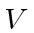times a positive exponential plus a negative exponential. As before,depends on position but we don't bother to write that for most of our calculations.

Putting this perturbation into the expression for, we getNote that the terms in the time integral will average to zero unless one of the exponents is nearly zero. If one of the exponents is zero, the amplitude to be in the state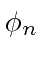will increase with time. To make an exponent zero we must have one of two conditions satisfied.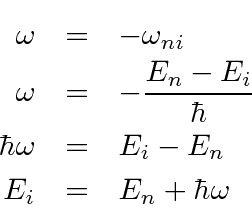This is energy conservation for the emission of a quantum of energy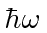.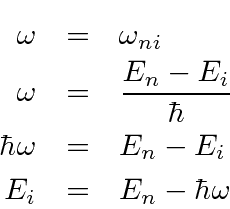This is energy conservation for the absorption of a quantum of energy. We can see the possibility of absorption of radiation or of stimulated emission.

For, the time integral of the exponential gives (some kind of) delta function of energy conservation. We will expend some effort to determine exactly what delta function it is.

Lets take the case of radiation of an energy quantum. If the initial and final states have energies such that this transition goes, the absorption term is completely negligible. (We can just use one of the exponentials at a time to make our formulas simpler.)

The amplitude to be in stateas a function of time is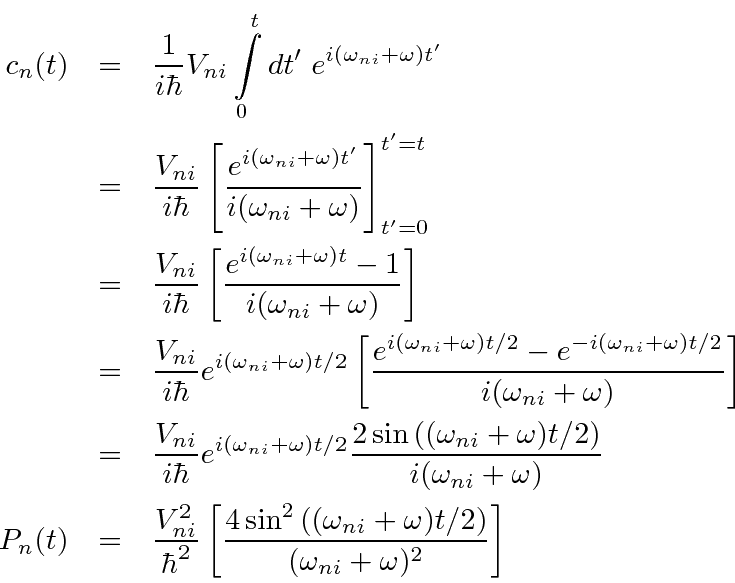In the last line above we have squared the amplitude to get the probability to be in the final state. The last formula is appropriate to use, as is, for short times. For long times (compared to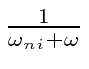which can be a VERY short time), the term in square brackets looks like some kind of delta function.

We will show that the quantity in square brackets in the last equation is. The probability to be in statethen isThe probability to be in the final stateincreases linearly with time. There is a delta function expressing energy conservation. The frequency of the harmonic perturbation must be set so thatis the energy difference between initial and final states. This is true both for the (stimulated) emission of a quantum of energy and for the absorption of a quantum.

Since the probability to be in the final state increases linearly with time, it is reasonable to describe this in terms of a transition rate. The transition rate is then given by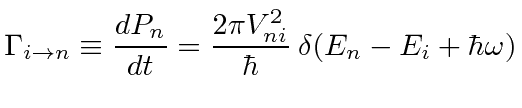We would get a similar result for increasing(absorbing energy) from the other exponential.It does not make a lot of sense to use this equation with a delta function to calculate the transition rate from a discrete state to a discrete state. If we tune the frequency just right we get infinity otherwise we get zero. This formula is what we need if either the initial or final state is a continuum state. If there is a free particle in the initial state or the final state, we have a continuum state. So, the absorption or emission of a particle, satisfies this condition.

The above results are very close to a transition rate formula known as Fermi's Golden Rule. Imagine that instead of one final statethere are a continuum of final states. The total rate to that continuum would be obtained by integrating over final state energy, an integral done simply with the delta function. We then have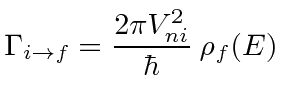where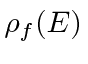is the density of final states. When particles (like photons or electrons) are emitted, the final state will be a continuum due to the continuum of states available to a free particle. We will need to carefully compute the density of those states, often known as phase space.

Jim Branson 2013-04-22